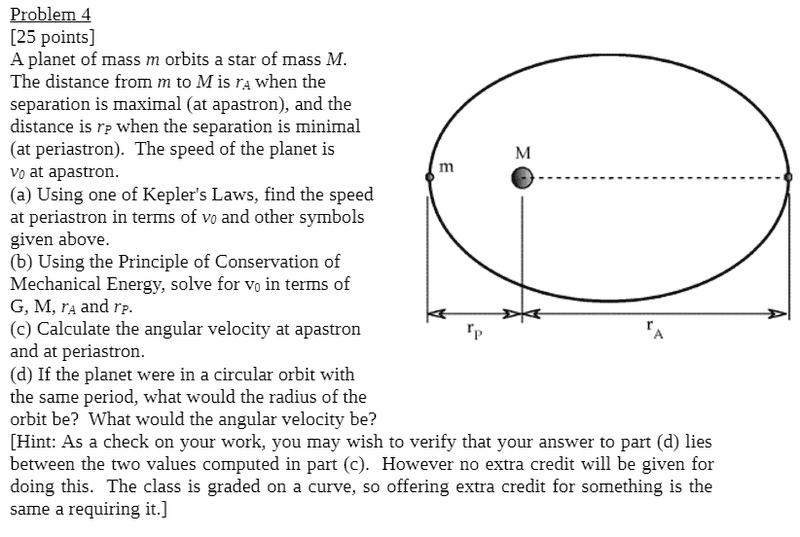# Kepler's Law for finding velocity

• Carpetfizz

## Homework Statement

[/B]
I need to help solving part a)## Homework Equations

[/B]
$$v= \frac{2\pi}{T}$$

$$(\frac{T_1}{T_2})^2 = (\frac{r_1}{r_2})^3$$

## The Attempt at a Solution

I'm not sure where to begin really. One approach I tried was getting T_1 in terms of v_0 and plugging it into Kepler's Third Law to get T_2, and plugging T_2 back into the velocity equation to get the new velocity. However, this did not match the given answer of:

$$v_{periastrom} = \frac{r_A}{r_P}v_0$$

There's only one orbit, so only one period. That rules out Kepler III as being of use here. What's left?

Kepler's second law? That each planet sweeps equal areas for equal periods of time? Not sure how to use that here.

Kepler's second law? That each planet sweeps equal areas for equal periods of time? Not sure how to use that here.
Yes. Kepler II is essentially a statement about the conservation of angular momentum. Consider a small time interval Δt and the areas swept out at the two locations over that small time interval. You might want to make a sketch using a bit of geometry to construct the approximations.

Okay so I have that $$\frac{dA}{dt} = 0.5*rvsin(\theta)$$ but I don't know how to use it to solve for v. I know that $$\frac{dA}{dt}$$ is constant though.

Go much simpler. Basic geometry. Draw the triangles that result assuming tangential velocities at the two locations. The areas of the triangles will approximate the areas swept out. If the Δt is small enough then these triangles will be a good approximation. In fact, as the Δt → 0, it's essentially the calculus approximation for the differential area element. What are the expressions for the areas of the two triangles?

Okay, so I have this so far:

$$A = \frac{1}{2}(t_1*v_0)r_A$$
$$A = \frac{1}{2}{t_2*v}r_P$$

but since I don't know the times I can't solve for v by setting them equal to each other.

Kepler II states equal areas in equal times. The times are identical.

Oh okay great, thank you so much!

When you write this up, use Δt to represent the time interval and make it clear that Δt is a small time interval (theoretically approaching zero). The geometric approximation using triangles to represent the areas swept out will fail if the time interval is large, as the triangle will not approximate the curvature of the ellipse.

•Carpetfizz